Anzeige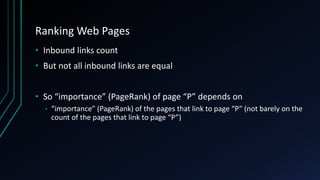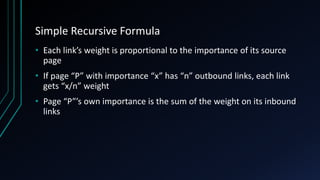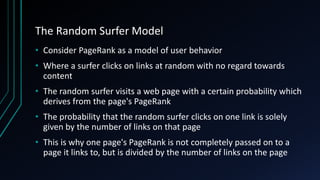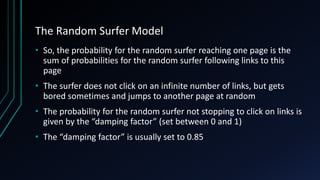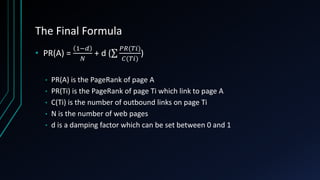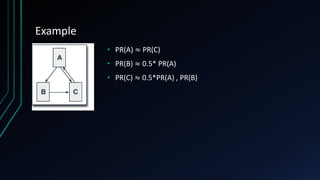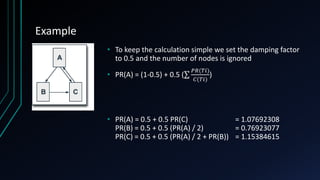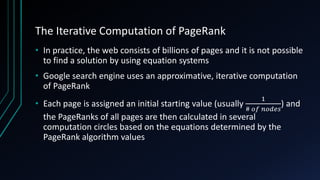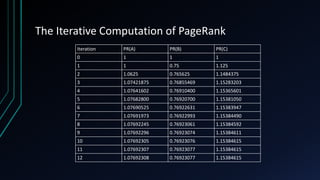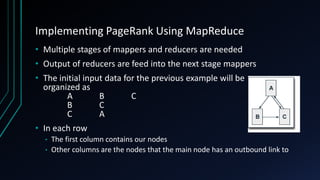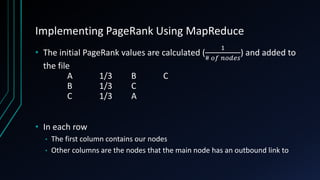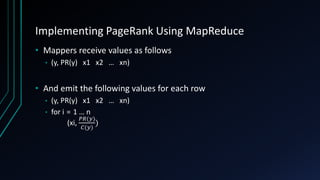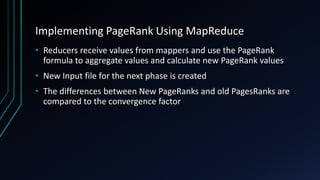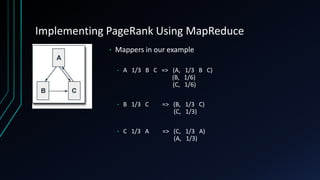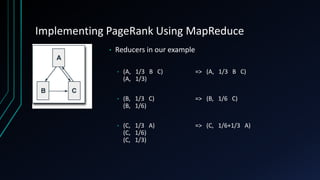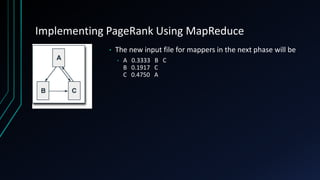1 von 22
Anzeige

### Implementing page rank algorithm using hadoop map reduce

1. Implementing PageRank Algorithm Using Hadoop MapReduce FARZAN HAJIAN FARZAN.HAJIAN@GMAIL.COM
2. Introduction • An algorithm for ranking web pages based on their importance • Developed by Lawrence Page and Sergey Brin (founders of Google) • Being used In Google to sort search results • Describes how probable web pages are to be visited by a random web surfer • It is an iterative graph processing algorithm
3. Ranking Web Pages • Web pages are not equally “Important” • www.amazon.com • www.my-personal-weblog.com • It is more likely that amazon.com is visited than the other web page • So it is more important (it has more weight) • WHY?
4. Ranking Web Pages • Inbound links count • The more inbound link a page has, the more important (probable to be visited) it become • Imagine two web pages • Page “A” (2 inbound links) • Page “B” (10 inbound links) • Which page is more important? • Page “B”
5. Ranking Web Pages • Now suppose this condition • Page “A” (2 inbound links) • amazon.com • facebook.com • Page “B” (10 inbound linked) • my-personal-weblog1.com • … • my-personal-weblog10.com • Now which page is more weighted?
6. Ranking Web Pages • Inbound links count • But not all inbound links are equal • So “importance” (PageRank) of page “P” depends on • “importance” (PageRank) of the pages that link to page “P” (not barely on the count of the pages that link to page “P”)
7. Simple Recursive Formula • Each link’s weight is proportional to the importance of its source page • If page “P” with importance “x” has “n” outbound links, each link gets “x/n” weight • Page “P”’s own importance is the sum of the weight on its inbound links
8. The Random Surfer Model • Consider PageRank as a model of user behavior • Where a surfer clicks on links at random with no regard towards content • The random surfer visits a web page with a certain probability which derives from the page's PageRank • The probability that the random surfer clicks on one link is solely given by the number of links on that page • This is why one page's PageRank is not completely passed on to a page it links to, but is divided by the number of links on the page
9. The Random Surfer Model • So, the probability for the random surfer reaching one page is the sum of probabilities for the random surfer following links to this page • The surfer does not click on an infinite number of links, but gets bored sometimes and jumps to another page at random • The probability for the random surfer not stopping to click on links is given by the “damping factor” (set between 0 and 1) • The “damping factor” is usually set to 0.85
10. The Final Formula • PR(A) = 1−𝑑 𝑁 + d ( 𝑃𝑅(𝑇𝑖) 𝐶(𝑇𝑖) ) • PR(A) is the PageRank of page A • PR(Ti) is the PageRank of page Ti which link to page A • C(Ti) is the number of outbound links on page Ti • N is the number of web pages • d is a damping factor which can be set between 0 and 1
11. Example • PR(A) ≈ PR(C) • PR(B) ≈ 0.5* PR(A) • PR(C) ≈ 0.5*PR(A) , PR(B)
12. Example • To keep the calculation simple we set the damping factor to 0.5 and the number of nodes is ignored • PR(A) = (1-0.5) + 0.5 ( 𝑃𝑅(𝑇𝑖) 𝐶(𝑇𝑖) ) • PR(A) = 0.5 + 0.5 PR(C) = 1.07692308 PR(B) = 0.5 + 0.5 (PR(A) / 2) = 0.76923077 PR(C) = 0.5 + 0.5 (PR(A) / 2 + PR(B)) = 1.15384615
13. The Iterative Computation of PageRank • In practice, the web consists of billions of pages and it is not possible to find a solution by using equation systems • Google search engine uses an approximative, iterative computation of PageRank • Each page is assigned an initial starting value (usually 1 # 𝑜𝑓 𝑛𝑜𝑑𝑒𝑠 ) and the PageRanks of all pages are then calculated in several computation circles based on the equations determined by the PageRank algorithm values
14. The Iterative Computation of PageRank Iteration PR(A) PR(B) PR(C) 0 1 1 1 1 1 0.75 1.125 2 1.0625 0.765625 1.1484375 3 1.07421875 0.76855469 1.15283203 4 1.07641602 0.76910400 1.15365601 5 1.07682800 0.76920700 1.15381050 6 1.07690525 0.76922631 1.15383947 7 1.07691973 0.76922993 1.15384490 8 1.07692245 0.76923061 1.15384592 9 1.07692296 0.76923074 1.15384611 10 1.07692305 0.76923076 1.15384615 11 1.07692307 0.76923077 1.15384615 12 1.07692308 0.76923077 1.15384615
15. Implementing PageRank Using MapReduce • Multiple stages of mappers and reducers are needed • Output of reducers are feed into the next stage mappers • The initial input data for the previous example will be organized as A B C B C C A • In each row • The first column contains our nodes • Other columns are the nodes that the main node has an outbound link to
16. Implementing PageRank Using MapReduce • The initial PageRank values are calculated ( 1 # 𝑜𝑓 𝑛𝑜𝑑𝑒𝑠 ) and added to the file A 1/3 B C B 1/3 C C 1/3 A • In each row • The first column contains our nodes • Other columns are the nodes that the main node has an outbound link to
17. Implementing PageRank Using MapReduce • Mappers receive values as follows • (y, PR(y) x1 x2 … xn) • And emit the following values for each row • (y, PR(y) x1 x2 … xn) • for i = 1 … n (xi, 𝑃𝑅(𝑦) 𝐶(𝑦) )
18. Implementing PageRank Using MapReduce • Reducers receive values from mappers and use the PageRank formula to aggregate values and calculate new PageRank values • New Input file for the next phase is created • The differences between New PageRanks and old PagesRanks are compared to the convergence factor
19. Implementing PageRank Using MapReduce • Mappers in our example • A 1/3 B C => (A, 1/3 B C) (B, 1/6) (C, 1/6) • B 1/3 C => (B, 1/3 C) (C, 1/3) • C 1/3 A => (C, 1/3 A) (A, 1/3)
20. Implementing PageRank Using MapReduce • Reducers in our example • (A, 1/3 B C) => (A, 1/3 B C) (A, 1/3) • (B, 1/3 C) => (B, 1/6 C) (B, 1/6) • (C, 1/3 A) => (C, 1/6+1/3 A) (C, 1/6) (C, 1/3)
21. Implementing PageRank Using MapReduce • The new input file for mappers in the next phase will be • A 0.3333 B C B 0.1917 C C 0.4750 A
22. Thank You
Anzeige